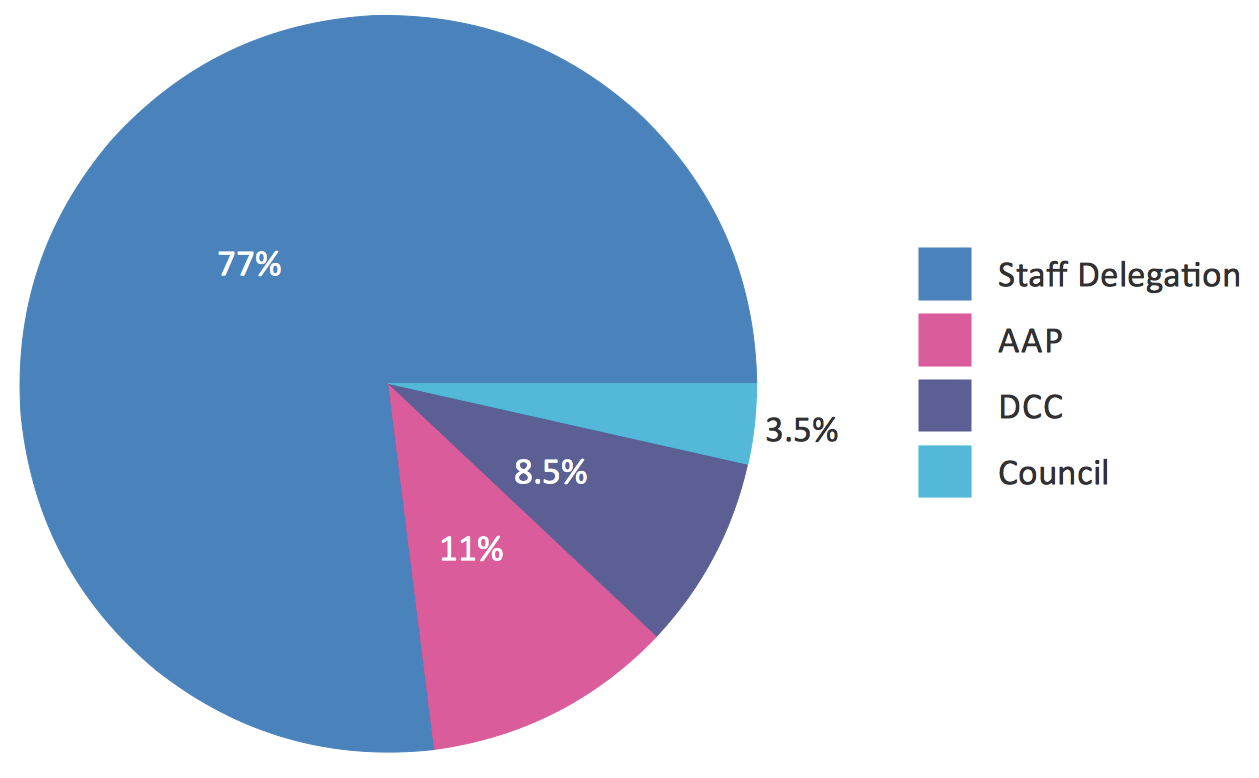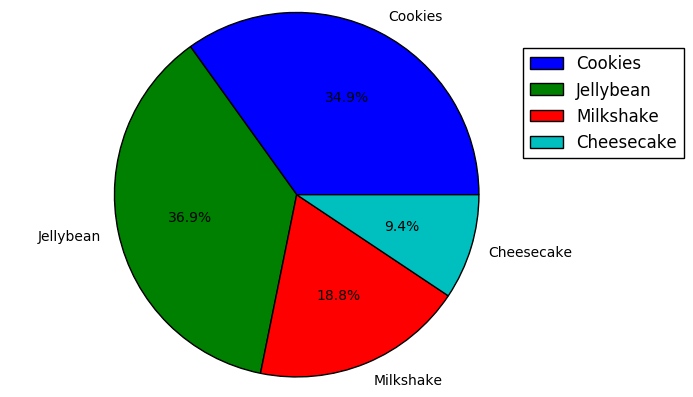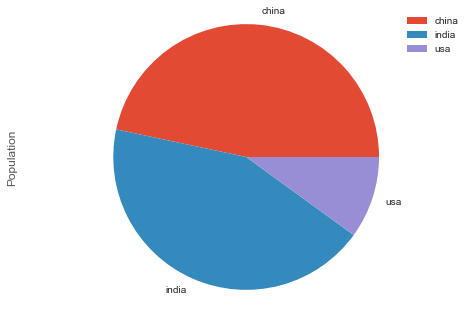#### 如何用python画饼图？

统计/机器学习 数据可视化 Python    浏览次数：4500        分享

0yayat   2018-02-21 20:46

##### 2个回答
5

labels = ['Cookies', 'Jellybean', 'Milkshake', 'Cheesecake']
sizes = [38.4, 40.6, 20.7, 10.3]
plt.pie(sizes, labels=labels, autopct='%1.1f%%')
plt.legend(loc=[0.9, 0.6])
plt.axis('equal')
plt.show()SofaSofa数据科学社区DS面试题库 DS面经Jiho   2018-02-22 22:46

3

import pandas as pd
import matplotlib.pyplot as plt
plt.style.use('ggplot')

df = pd.DataFrame({'Country': ['china', 'india', 'usa'], 'Population': [14, 13, 3]})

df.Population.groupby(df.Country).sum().plot(kind='pie')
plt.axis('equal')
plt.legend()
plt.show()SofaSofa数据科学社区DS面试题库 DS面经数据痴汉   2019-05-12 08:45相关主题